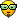# Force object to move on Y axes don't work

I think it a bug.

If I want to force an object to move left or right on the X axes, I would use object.ForceX() and it works.
But if want to force an object to move up or down on the Y axes using object.ForceY() it doesn’t work, the object moves on the X axes instead of Y.

I have uploaded an example to here:

Where are you using that functions, c++ code?
I only use this values as properties variables (I don’t know you use them to add a force), they return the “Average X coordinates of forces” or Y coordinates, is the returned value the bug?

EDIT: Ohhh, I’m testing you Project.gdg(I’m sorry)
This is not good, you are using a force as angle. The Object.ForceY() return the forces in the Y-axis of the object (Object.ForceY() = 0, because the object doesn’t move in y-axis), so the object move in angle = 0 (at right).
To move up and down you have to put 270 and 90 (respectively) in Angle.

Am, but the expression editor dropped me this code to use as the Y coordinates of the forceWhat I understand, this command should set the coordinates of the angle to Y axes and I can set the actual direction on the axes by using positive or negative value in Length.
It works on X axes if I use object.ForceX(). But maybe I misunderstand what " Y coordinates of Force " means, my English is not perfect especially the grammar.

But if I use only an integer it worksThanks.

You can use the Object.ForceX() and Object.ForceY(), and, of course, they appear in the Expression Editor, but you have to identify their “meanings”, you could use as Angle the width of the camera 0, but it has not sense (I don’t know if you understand me):
Object.ForceX() = The amount of all forces in the X-axis of the object.
Object.ForceY() = The amount of all forces in the Y-axis of the object.

If you have a Ball object, with mass, and you jump at right with the ball:
Ball.ForceX() = The X-component of the jump force (positive because the ball jump at right)
Ball.ForceY() = The Y-component of the jump force + The gravity force (negative when the ball is jumping, positive when it’s falling)When you start you game the tank doesn’t move, so Tank.ForceX() = 0 and Tank.ForceY() = 0
Then you add a force with angle = Tank.ForceX() or angle = Tank.ForceY(), angle = 0 and your Tank will move at right (angle = 0)
Then Tank.ForceX() = 0 and Tank.ForceY() = 0, because you use damping = 0 (this meaning: after apply the force, this become *0 = 0)

Try to set damping = 0.1 and Tank.ForceX() as angle, and your test will crash in a strange Y-force (because the angle will be > 0, because the damping > 0 and Tank.ForceX() != 0)

Because the integer is the angle, you can use any number (integer or float), and will work, but you should try this means somethingHere is an image that shows the GD’s direction system (from GD wiki):

Thanks Lizard, you helped a lotIt makes everything more clear especially that image. Maybe I should spend some time to read through everything on the wiki before I go any further with GDThe image?
I’ve noticed that people undersand me better with images, I try to use them a lotMaybe not everything, but I recommend it.